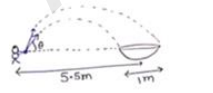# One man is sitting on the shores of a river.`
Question:

One man is sitting on the shores of a river. He is in the line of $1.0 \mathrm{~m}$ long boat and is $5.5 \mathrm{~m}$ away from the center of the boat. If he can throw the apply only with a speed of $10 \mathrm{~m} / \mathrm{s}$. find the minimum and maximum angles of projection for successful shot. Assume that the point of projection and the edge pf the boat are in the same horizontal level.

Solution:

For near point of boat

$\mathrm{R}=5 \mathrm{~m}=\frac{\mathrm{u}^{\mathrm{m}} \sin 2 \theta}{\mathrm{g}} \mathrm{g}$

$5=\frac{(10)^{2} \sin 2 e}{g}$$\theta=15^{\circ}$ or $75^{\circ}$

For for point of boat

$\mathrm{R}=6 \mathrm{~m}=\frac{\mathrm{u}^{\mathrm{a}^{\mathrm{s}} \sin 2 \theta}}{\mathrm{g}}$

$\theta=18^{\circ}$ or $71^{\circ}$

For a successful shot angle may vary from $15^{\circ}$ to $18^{\circ}$ or $71^{\circ}$ to $75^{\circ}$

Minimum angle $=15^{\circ}$

Maximum angle $=75^{\circ}$